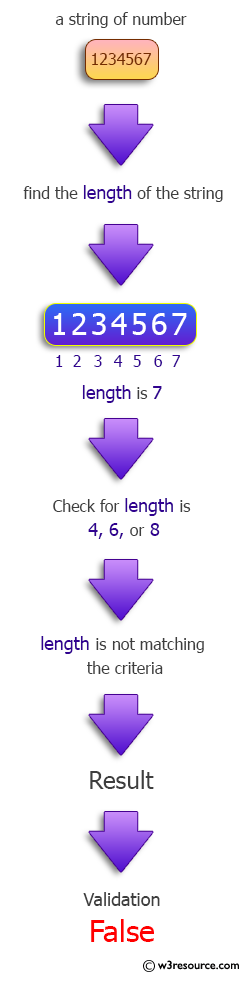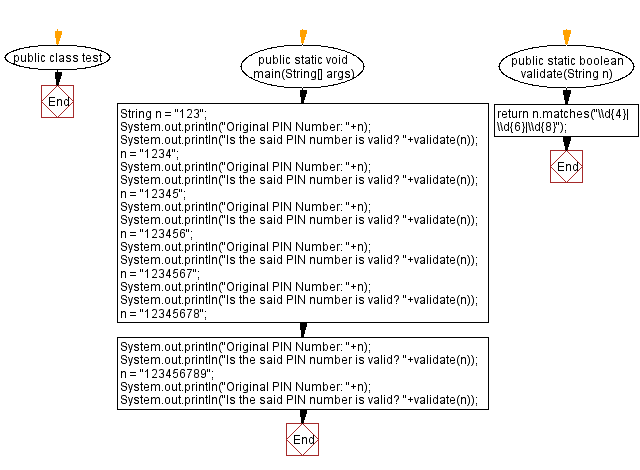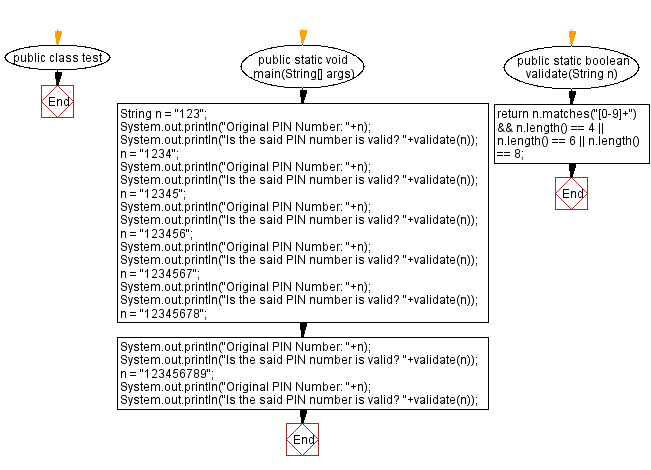﻿ Java: Validate a personal identification number (PIN)# Java programming Exercises: Validate a personal identification number (PIN)

## Java Regular Expression: Exercise-18 with Solution

(PIN): A personal identification number, or PIN, is a string of at least four digits used to unlock a bank account or card to which it has been assigned.

Write a Java program to validate a personal identification number (PIN). Assume the length of a PIN number is 4, 6 or 8.

Sample Solution-1:

Java Code:

``````public class test {

public static void main(String[] args) {
String n = "123";
System.out.println("Original PIN Number: "+n);
System.out.println("Is the said PIN number is valid? "+validate(n));
n = "1234";
System.out.println("Original PIN Number: "+n);
System.out.println("Is the said PIN number is valid? "+validate(n));
n = "12345";
System.out.println("Original PIN Number: "+n);
System.out.println("Is the said PIN number is valid? "+validate(n));
n = "123456";
System.out.println("Original PIN Number: "+n);
System.out.println("Is the said PIN number is valid? "+validate(n));
n = "1234567";
System.out.println("Original PIN Number: "+n);
System.out.println("Is the said PIN number is valid? "+validate(n));
n = "12345678";
System.out.println("Original PIN Number: "+n);
System.out.println("Is the said PIN number is valid? "+validate(n));
n = "123456789";
System.out.println("Original PIN Number: "+n);
System.out.println("Is the said PIN number is valid? "+validate(n));
}

public static boolean validate(String n) {
return n.matches("\\d{4}|\\d{6}|\\d{8}");
}
}
```
```

Sample Output:

```Original PIN Number: 123
Is the said PIN number is valid? false
Original PIN Number: 1234
Is the said PIN number is valid? true
Original PIN Number: 12345
Is the said PIN number is valid? false
Original PIN Number: 123456
Is the said PIN number is valid? true
Original PIN Number: 1234567
Is the said PIN number is valid? false
Original PIN Number: 12345678
Is the said PIN number is valid? true
Original PIN Number: 123456789
Is the said PIN number is valid? false
```

Pictorial Presentation:Flowchart :Sample Solution-2:

Java Code:

``````public class test {

public static void main(String[] args) {
String n = "123";
System.out.println("Original PIN Number: "+n);
System.out.println("Is the said PIN number is valid? "+validate(n));
n = "1234";
System.out.println("Original PIN Number: "+n);
System.out.println("Is the said PIN number is valid? "+validate(n));
n = "12345";
System.out.println("Original PIN Number: "+n);
System.out.println("Is the said PIN number is valid? "+validate(n));
n = "123456";
System.out.println("Original PIN Number: "+n);
System.out.println("Is the said PIN number is valid? "+validate(n));
n = "1234567";
System.out.println("Original PIN Number: "+n);
System.out.println("Is the said PIN number is valid? "+validate(n));
n = "12345678";
System.out.println("Original PIN Number: "+n);
System.out.println("Is the said PIN number is valid? "+validate(n));
n = "123456789";
System.out.println("Original PIN Number: "+n);
System.out.println("Is the said PIN number is valid? "+validate(n));
}

public static boolean validate(String n) {
return n.matches("[0-9]+") && n.length() == 4 || n.length() == 6 || n.length() == 8;
}
}
```
```

Sample Output:

```Original PIN Number: 123
Is the said PIN number is valid? false
Original PIN Number: 1234
Is the said PIN number is valid? true
Original PIN Number: 12345
Is the said PIN number is valid? false
Original PIN Number: 123456
Is the said PIN number is valid? true
Original PIN Number: 1234567
Is the said PIN number is valid? false
Original PIN Number: 12345678
Is the said PIN number is valid? true
Original PIN Number: 123456789
Is the said PIN number is valid? false
```

Flowchart :Java Code Editor:

Next: Remove the specific letters from a string and return the new string.

What is the difficulty level of this exercise?

Test your Programming skills with w3resource's quiz.

﻿

## Java: Tips of the Day

Split Java String by New Line

```String lines[] = string.split("\\r?\\n");
```

There's only really two newlines (UNIX and Windows) that you need to worry about.

Ref: https://bit.ly/3dHk7ip